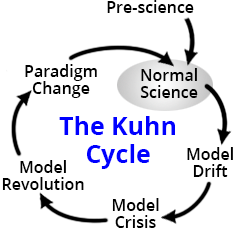# Which problems make good research problems?

Sabine Hossenfelder in Back Reaction:Scientists solve problems; that’s their job. But which problems are promising topics of research? This is the question I set out to answer in Lost in Math at least concerning the foundations of physics.

A first, rough, classification of research problems can be made using Thomas Kuhn’s cycle of scientific theories. Kuhn’s cycle consists of a phase of “normal science” followed by “crisis” leading to a paradigm change, after which a new phase of “normal science” begins. This grossly oversimplifies reality, but it will be good enough for what follows.

During the phase of normal science, research questions usually can be phrased as “How do we measure this?” (for the experimentalists) or “How do we calculate this?” (for the theorists).

In the foundations of physics, we have a lot of these “normal problems.” For the experimentalists it’s because the low-hanging fruits have been picked and measuring anything new becomes increasingly challenging. For the theorists it’s because in physics predictions don’t just fall out of hypotheses. We often need many steps of argumentation and lengthy calculations to derive quantitative consequences from a theory’s premises.

A good example for a normal problem in the foundations of physics is cold dark matter. The hypothesis is easy enough: There’s some cold, dark, stuff in the cosmos that behaves like a fluid and interacts weakly both with itself and other matter. But that by itself isn’t a useful prediction. A concrete research problem would instead be: “What is the effect of cold dark matter on the temperature fluctuations of the cosmic microwave background?” And then the experimental question “How can we measure this?”

Other problems of this type in the foundations of physics are “What is the gravitational contribution to the magnetic moment of the muon?,” or “What is the photon background for proton scattering at the Large Hadron Collider?”

Answering such normal problems expands our understanding of existing theories. These are calculations that can be done within the frameworks we have, but calculations can be be challenging.

The examples in the previous paragraphs are solved problems, or at least problems that we know how to solve, though you can always ask for higher precision. But we also have unsolved problems in this category.

More here.